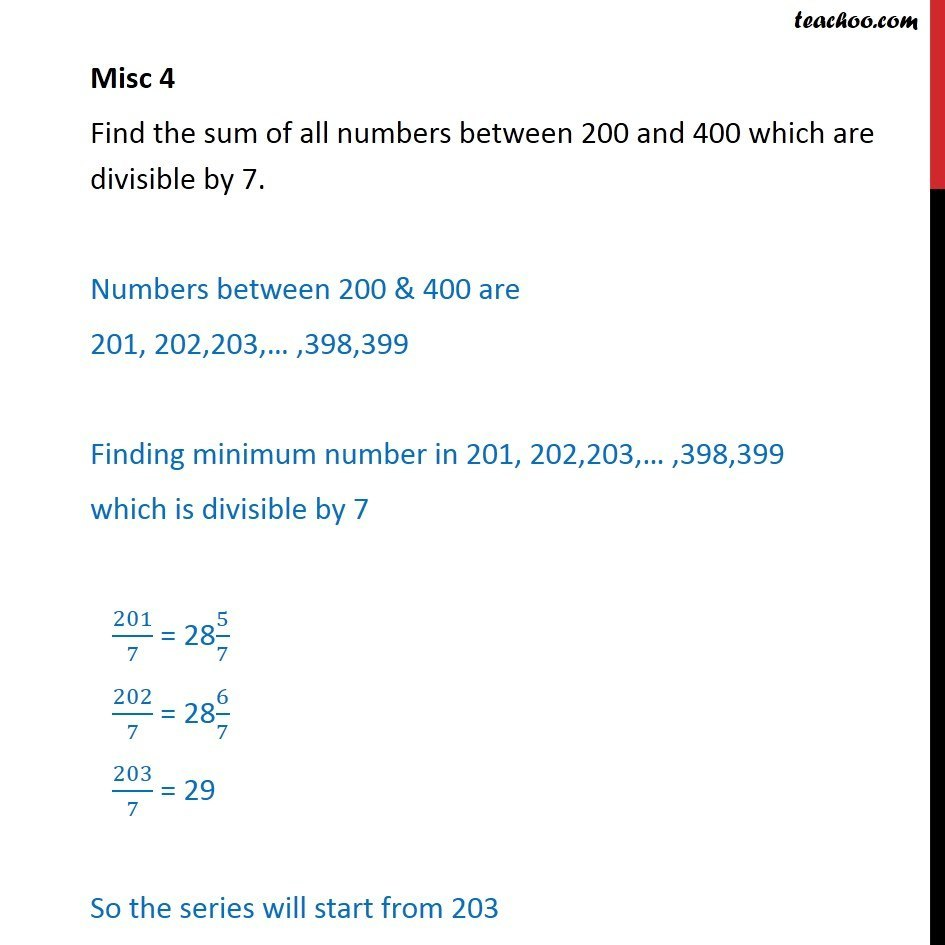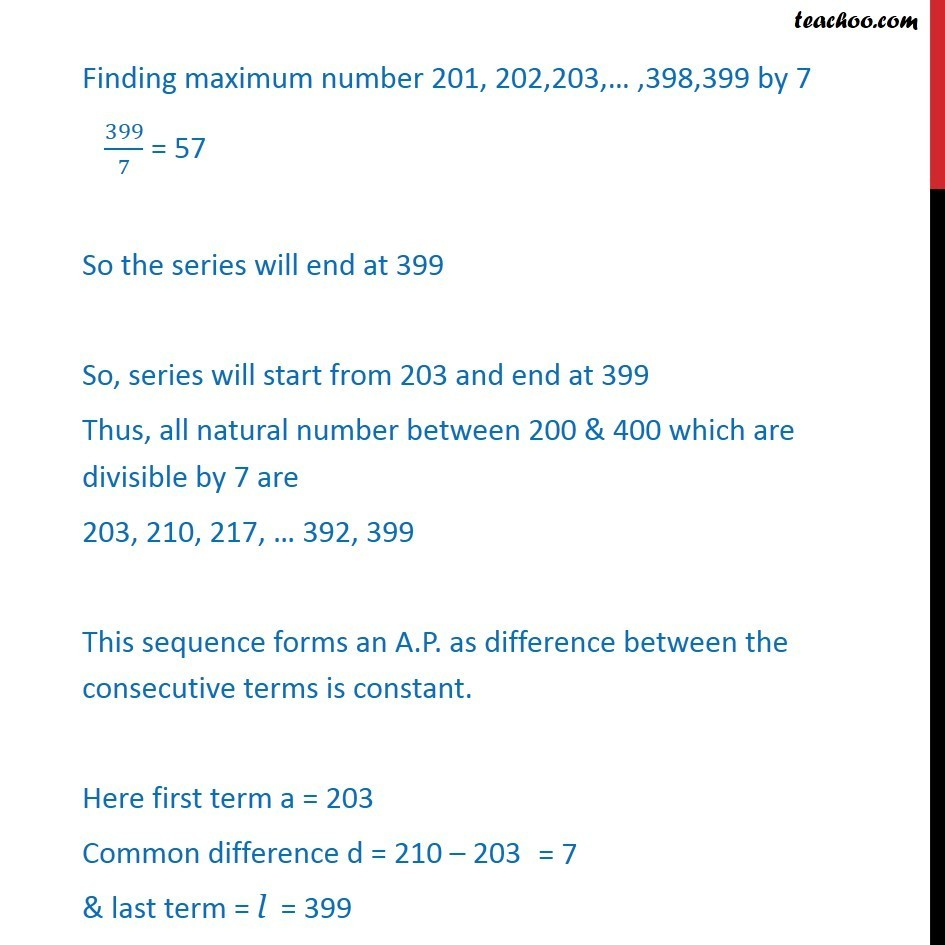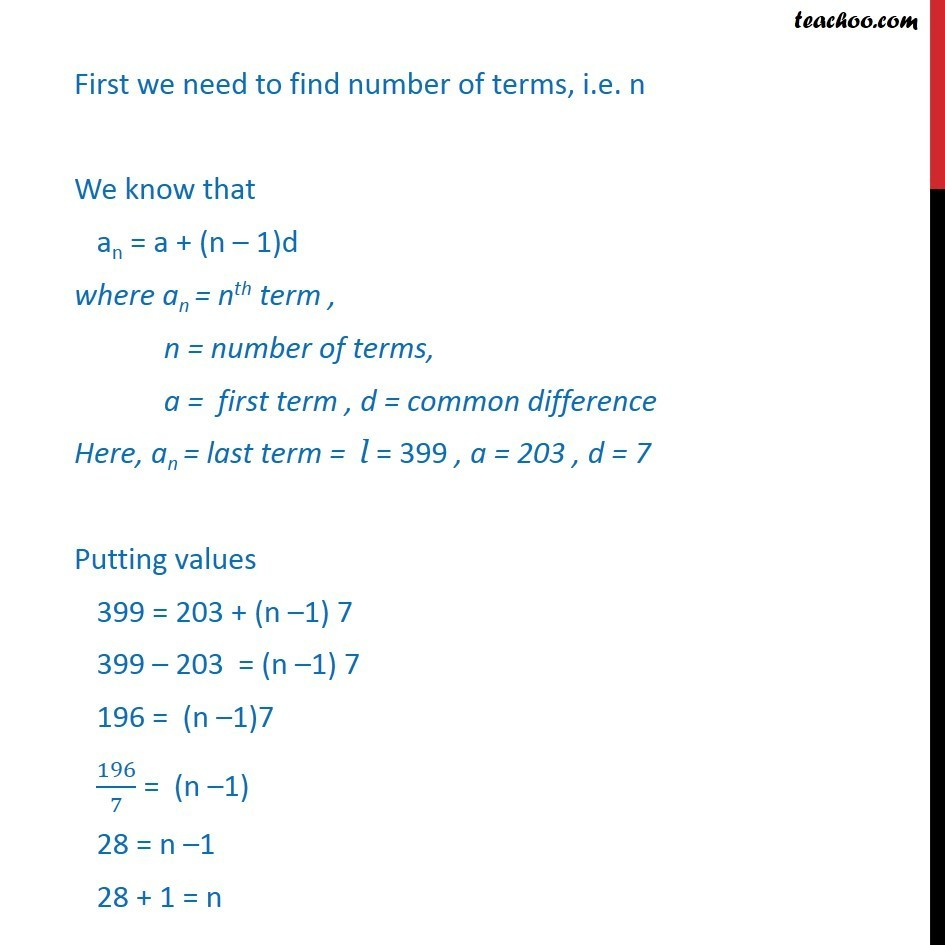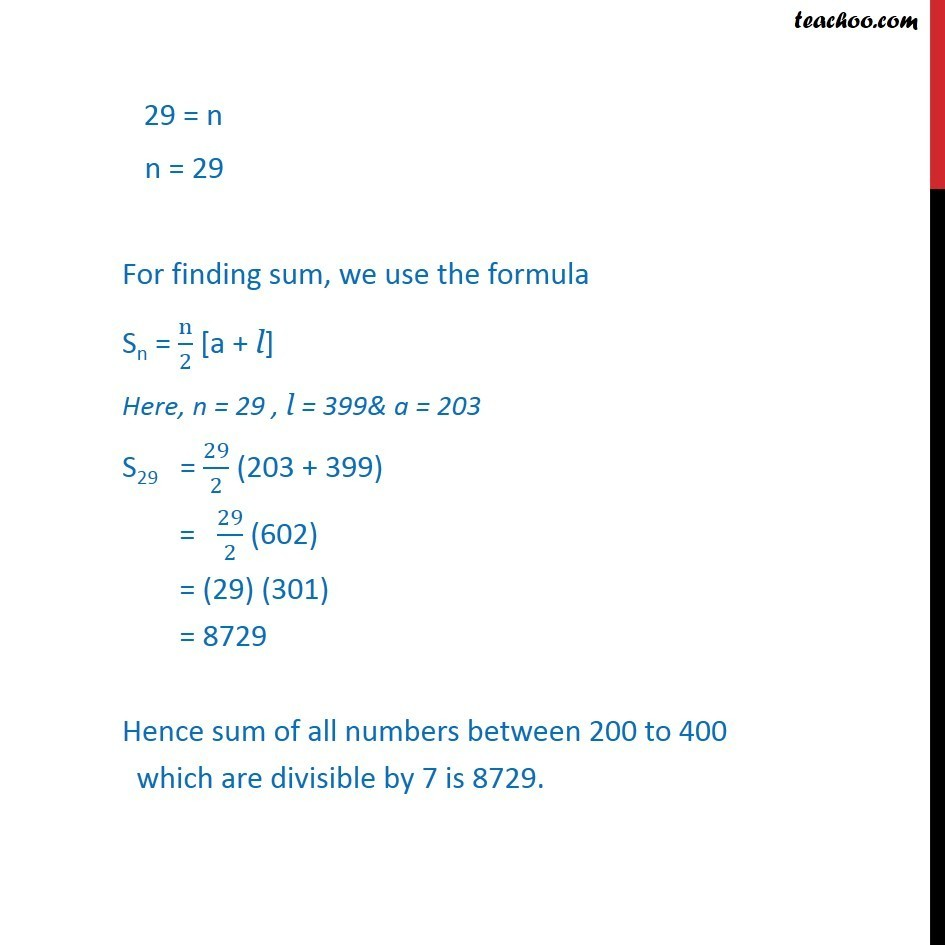Miscellaneous

Chapter 8 Class 11 Sequences and Series
Serial order wiseLearn in your speed, with individual attention - Teachoo Maths 1-on-1 Class

### Transcript

Question 4 Find the sum of all numbers between 200 and 400 which are divisible by 7. Numbers between 200 & 400 are 201, 202,203,… ,398,399 Finding minimum number in 201, 202,203,… ,398,399 which is divisible by 7 201/7 = 285/7 202/7 = 286/7 203/7 = 29 So the series will start from 203 Finding maximum number 201, 202,203,… ,398,399 by 7 399/7 = 57 So the series will end at 399 So, series will start from 203 and end at 399 Thus, all natural number between 200 & 400 which are divisible by 7 are 203, 210, 217, ­­­­­­­­… 392, 399 This sequence forms an A.P. as difference between the consecutive terms is constant. Here first term a = 203 Common difference d = 210 – 203 & last term = l = 399 First we need to find number of terms, i.e. n We know that an = a + (n – 1)d where an = nth term , n = number of terms, a = first term , d = common difference Here, an = last term = l = 399 , a = 203 , d = 7 Putting values 399 = 203 + (n –1) 7 399 – 203 = (n –1) 7 196 = (n –1)7 196/7 = (n –1) 28 = n –1 28 + 1 = n 29 = n n = 29 For finding sum, we use the formula Sn = n/2 [a + l] Here, n = 29 , l = 399& a = 203 S29 = 29/2 (203 + 399) = 29/2 (602) = (29) (301) = 8729 Hence sum of all numbers between 200 to 400 which are divisible by 7 is 8729.